## Perimeter of Squares and Rectangles

Practice Unlimited Questions

#### What is the perimeter of a Rectangle?

The perimeter of a rectangle is the length of all its 4 sides. In other words, the perimeter of a rectangle is the total distance around it.

Formula to calculate the perimeter of a rectangle is:

Perimeter of Rectangle  =  2  ×  Length  +  2  ×  Breadth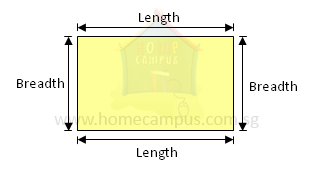The perimeter can be represented using a model as below.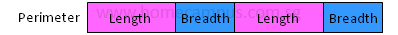=  2  ×  Length  +  2  ×  Breadth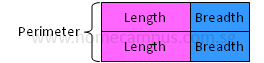Length  +  Breadth  =  Perimeter  ÷  2

#### What is the perimeter of a Square?

Perimeter of Square  =  4  ×  Length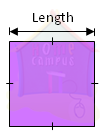Property of a square: All sides are equal.
Perimeter  =  Length  +  Length  +  Length  +  Length
=  4  ×  Length

#### 1. What is the perimeter of a rectangle of sides 4 cm by 3 cm?Perimeter of rectangle
=  4 cm  +  3 cm  +  4 cm  +  3 cm
=  14 cm

The perimeter of the rectangle is 14 cm.
The perimeter of a rectangle is the total distance around it.

#### 2. What is the perimeter of a square of side 4 cm?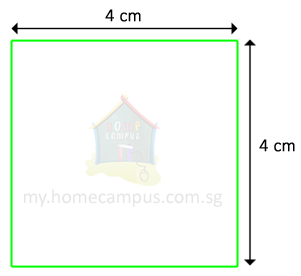Perimeter of square
=  4 cm  +  4 cm  +  4 cm  +  4 cm
=  16 cm

The perimeter of the square is 16 cm.
The perimeter of a square is the total distance around it.

#### 3. Find the perimeter of each figure below. What can you tell about their perimeter?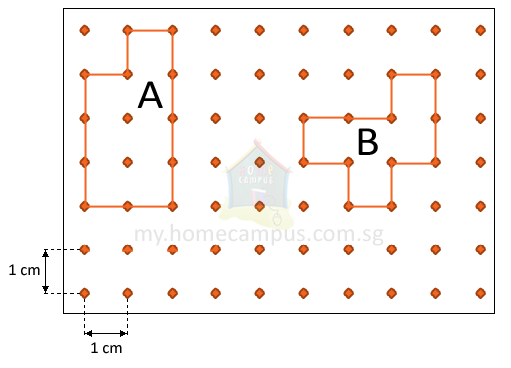The perimeter of a figure is the total distance around it.

Perimeter of figure A
=  1 cm  +  1 cm  +  1 cm  +  4 cm  +  2 cm  +  3 cm
=  12 cm

Perimeter of figure B
=  2 cm  +  1 cm  +  1 cm  +  2 cm  +  1 cm  +  1 cm  +  1 cm  +  1 cm  +  1 cm  +  1 cm
=  12 cm

The perimeter of each figure is 12 cm.
Two different figures can have the same perimeter.